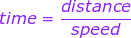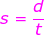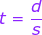## 6.SP.1-6.SP.5 Statistics and Probability

Posted: February 26, 2018 in Advance, Periods 1&2, Periods 3&46.SP.A.1Recognize a statistical question as one that anticipates variability in the data related to the question and accounts for it in the answers.

Lessons:

Isolation Drills:

TenMarks Assignment:

• 6.SP.1 Recognizing Statistical Questions

6.SP.A.2 Understand that a set of data collected to answer a statistical question has a distribution which can be described by its center, spread, and overall shape.

Lessons:

Isolation Drills:

TenMarks Assignment:

• 6.SP.2 Describe Data Distribution: Use Center/Spread/Shape

6.SP.A.3 Recognize that a measure of center for a numerical data set summarizes all of its values with a single number, while a measure of variation describes how its values vary with a single number.

Lessons:

Isolation Drills:

TenMarks Assignment:

•  6.SP.3 Recognize Measures of Center & Measures of Variation

6.SP.B.4Display numerical data in plots on a number line, including dot plots, histograms, and box plots.

TenMarks Assignment:

• 6.SP.4 Displaying Numerical Data

6.SP.B.5 Summarize numerical data sets in relation to their context Reporting the number of observations and describing the nature of the attribute under investigation, including how it was measured and its units of measurement. Giving quantitative measures of center (median and/or mean) and variability (interquartile range and/or mean absolute deviation), as well as describing any overall pattern and any striking deviations from the overall pattern with reference to the context in which the data were gathered.  Relating the choice of measures of center and variability to the shape of the data distribution and the context in which the data were gathered.

Lessons:

Isolation Drills:

TenMarks Assignment:

• 6.SP.5a Identify: Observation Numbers Based on a Data Display
•  6.SP.5b Attributes of Data Displays
• 6.SP.5c Find: Measures of Center and Variability of Data Sets
• 6.SP.5d Measures of Center and Variability: Shape Data Display

## 7.SP.1-7.SP.4 Syllabus

Standards:

7.SP.A.1Understand that statistics can be used to gain information about a population by examining a sample of the population; generalizations about a population from a sample are valid only if the sample is representative of that population. Understand that random sampling tends to produce representative samples and support valid inferences.

7.SP.A.2 Use data from a random sample to draw  inferences about a population with an unknown characteristic of interest. Generate multiple samples (or simulated samples) of the same size to gauge the variation in estimates or predictions.

7.SP.B.3 Informally assess the degree of visual overlap of two numerical data distributions with similar variabilities, measuring the difference between the centers by expressing it as a multiple of a measure of variability.

7.SP.B.4 Use measures of center and measures of variability for numerical data from random samples to draw informal comparative inferences about two populations.

Lesson Videos:

### Data, statistics, and probability

Isolation Drills:

TenMarks:

7.SP.1
Understanding and Inferring Sample Data

7.SP.2
Inferring Variation in Data Samples
7.SP.3
Understanding Overlap of Data Distributions
7.SP.4
Using Measures of Center to Draw Inferences
7.SP.4
Measures of Center and Variability to Draw Inferences

## The Meatball Challenge

Posted: November 19, 2017 in Advance, Periods 1&2, Periods 3&4, Sports Science MATH

6.RP.A.1

Understand the concept of a ratio and use ratio language to describe a ratio relationship between two quantities.

6.RP.A.2

Understand the concept of a unit rate a/b associated with a ratio a:b with b ≠ 0, and use rate language in the context of a ratio relationship.

6.RP.A.3

Use ratio and rate reasoning to solve real-world and mathematical problems, e.g., by reasoning about tables of equivalent ratios, tape diagrams, double number line diagrams, or equations.

6.RP.A.3a

Make tables of equivalent ratios relating quantities with whole-number measurements, find missing values in the tables, and plot the pairs of values on the coordinate plane. Use tables to compare ratios.

6.RP.A.3b

Solve unit rate problems including those involving unit pricing and constant speed.

## Gateway Arch turns 51

Posted: November 7, 2017 in Advance, Periods 1&2, Periods 3&4

#### Natalie Morales pops out the top of the Gateway Arch9,000 visitors a year.

4 minute ride up and 3 minute ride down.

6.RP.A.1
Understand the concept of a ratio and use ratio language to describe a ratio relationship between two quantities.

6.RP.A.2

Understand the concept of a unit rate a/b associated with a ratio a:b with b ≠ 0, and use rate language in the context of a ratio relationship.

6.RP.A.3

Use ratio and rate reasoning to solve real-world and mathematical problems, e.g., by reasoning about tables of equivalent ratios, tape diagrams, double number line diagrams, or equations.

6.RP.A.3a

Make tables of equivalent ratios relating quantities with whole-number measurements, find missing values in the tables, and plot the pairs of values on the coordinate plane. Use tables to compare ratios.

## Speed Project

Posted: October 26, 2017 in Periods 1&2, Periods 3&4• 6.NS.1 – Dividing Two Fractions
• 6.NS.1 – Dividing Fractions and Mixed Numbers
• 6.NS.1 – Dividing Fractions and Whole Numbers
• 6.NS.2 – Dividing Multi-Digit Numbers
• 6.NS.3 – Estimate All Operation Answers: Multi-Digit Decimals
• 6.NS.3 – Add/Subtract/Multiply/Divide: Multi-Digit Decimals

### M = (Distance / Time) * (3,600 / 5,280)

Distance measured in Feet

Time recorded in Seconds

3600 is the number of Seconds in an Hour

5280 is the number of Feet in a Mile

## Sport Science: Paul Rabil Lacrosse Shot,,,,## (6.NS.5-6.NS.8) Number System Fluency Syllabus

Posted: October 24, 2017 in Advance, Periods 1&2, Periods 3&4

Understand the relationship between positive and negative numbers (6.NS.C.5)

• I can explain how positive and negative numbers relate to real world examples and the meaning of zero in each case.

Lessons:

Relate positive and negative quantities

In this lesson you will learn how positive and negative quantities are related by looking at some different real-world examples.

• Created By: Suzanne Geltman
• Standards:6. NS.C.5

Relate positive and negative quantities; apply to elevation

In this lesson you will learn how positive and negative numbers are used in elevation by looking at distances above and below sea level.

• Created By: Suzanne Geltman
• Standards:6. NS.C.5

Relate positive and negative quantities; apply to temperature

In this lesson you will learn how positive and negative numbers are used in temperature by looking at temperatures above and below freezing.

• Created By: Suzanne Geltman
• Standards: 6.NS.C.5

Relate positive and negative quantities; apply to bank balance

In this lesson you will learn how positive and negative numbers are used with money by looking at bank accounts with greater or less than \$0.

• Created By: Suzanne Geltman
• Standards: 6.NS.C.5

Understand rational numbers and ordered pairs; place pairs of rational numbers on the coordinate plane (6.NS.C.6)

• I can explain how each rational number has an opposite value.
• I can explain how each rational number has an opposite value.
• I can explain how the signs of any ordered pair change in each quadrant of the coordinate graph
• I can explain how changing the sign of the numbers in an ordered pair causes it to reflex on one or both axes
• I can locate a rational number on the number line.
• I can plot an ordered pair of rational numbers on the coordinate plane.

Lessons:

Understand the coordinate plane as horizontal and vertical number lines

In this lesson you will learn about the coordinate plane by combining horizontal and vertical number lines.

• Created By: Christine Hansen
• Standards: 6.NS.C.6b

Graph points on a coordinate plane

In this lesson you will learn how to graph points by using a coordinate plane.

• Created By: Christine Hansen
• Standards: 6.NS.C.6b

Graph rational numbers on a coordinate plane

In this lesson you will learn how to graph rational numbers by using a coordinate plane.

• Created By: Christine Hansen
• Standards: 6.NS.C.6c

Reflect points over the x and y axes

In this lesson you will learn how to reflect points over the x and y axes by using a coordinate plane.

• Created By: Christine Hansen
• Standards: 6.NS.C.6b

Translate shapes across the x and y axes

In this lesson you will learn how to translate shapes across the x and y axes by using a coordinate plane.

• Created By: Christine Hansen
• Standards: 6.NS.C.6b

Find the value of a number using its distance and direction from zero

In this lesson you will learn how to find the value of a number by comparing its distance and direction from 0.

• Created By: James Morrison
• Standards: 6.NS.C.6b, 6.NS.C.6c

Find opposite numbers on the number line

In this lesson you will learn how to find opposite numbers by comparing their distances and directions from 0.

• Created By: James Morrison
• Standards: 6.NS.C.6a, 6.NS.C.6b, 6.NS.C.6c

Locate a point on the coordinate plane

In this lesson you will learn how to locate a point on a coordinate plane by plotting an ordered pair.

• Created By: James Morrison
• Standards: 6.NS.C.6b, 6.NS.C.6c

Determine the quadrant of a point

In this lesson you will learn how to determine the quadrant of a point by examining the values of an ordered pair.

• Created By: James Morrison
• Standards: 6.NS.C.6b, 6.NS.C.6c

Predict the reflection of a point by changing values

In this lesson you will learn how to predict the reflection of a point by changing one or more of its values in an ordered pair.

• Created By: James Morrison
• Standards:6. NS.C.6b, 6.NS.C.6c

Understand the opposite of a number by looking at a number line

In this lesson you will learn about the opposite of a number by looking at a number line.

• Created By: Christine Hansen
• Standards: 6.NS.C.6a

Understand the opposites of fractions by looking at a number line

In this lesson you will learn about the opposites of fractions by looking at a number line.

• Created By: Christine Hansen
• Standards: 6.NS.C.6a

Rewrite a fraction as a decimal using division

In this lesson you will learn to rewrite a fraction as a decimal by using division.

• Created By: Christine Hansen
• Standards: 6.NS.C.6a

Rewrite a fraction as a repeating decimal using division

In this lesson you will learn to rewrite a fraction as a decimal by using division.

• Created By: Christine Hansen
• Standards: 6.NS.C.6c

Rewrite decimals as fractions by using equivalent fractions

In this lesson you will learn to rewrite a decimal as a fraction by using equivalent fractions.

• Created By: Christine Hansen
• Standards: 6.NS.C.6a

Locate positive rational numbers using a number line

In this lesson you will learn about locating positive rational numbers by using a number line.

• Created By: Christine Hansen
• Standards: 6.NS.C.6a

Locate rational numbers using a number line

In this lesson you will learn about locating rational numbers by using a number line.

• Created By: Christine Hansen
• Standards: 6.NS.C.6c

Interpret statements of inequality and write, interpret, and explain statements of order for rational numbers (6.NS.C.7)

• I can compare two rational numbers on a number line and in an inequality.
• I can explain the meaning of ordering rational numbers in a real world situation.
• I can explain how absolute value of a number relates to its distance from zero.
• I can explain how the absolute value of a number is used in real world situations to show magnitude.
• I can compare how the absolute value of a number relates to value of the number on the number line.

Lessons:

Understand the relationship between two numbers using a number line

In this lesson you will learn about the relationship between two numbers by finding their location on a number line.

• Created By: Timothy Hays
• Standards: 6.NS.C.7a

Understand the relationship between two negative numbers using a number lines

In this lesson you will learn about the relationship between two negative numbers by finding their location on a number line.

• Created By: Timothy Hays
• Standards: 6.NS.C.7a

Compare two positive or negative numbers in real-world situations

In this lesson you will learn about comparing two positive or negative numbers in real-world situations by using a number line.

• Created By: Timothy Hays
• Standards:6. NS.C.7b

Compare more than two positive or negative numbers in real-world situations

In this lesson you will learn about comparing more than two positive or negative numbers in real-world situations by using a number line.

Find absolute value using a number line

In this lesson you will learn how to find the absolute value of a number by using a number line.

• Created By: Timothy Hays
• Standards: 6.NS.C.7c

Use a number line to understand the relationship between rational numbers and absolute value

In this lesson you will learn about the relationship between rational numbers and absolute value by using a number line.

• Created By: Timothy Hays
• Standards: 6.NS.C.7c

Describe negative values with words

In this lesson you will learn to describe negative values with words by using a number line.

• Created By: Timothy Hays
• Standards: 6.NS.C.7c

Interpret absolute value in real-world situations

In this lesson you will learn to interpret absolute value in real-world situations by using a number line.

• Created By: Timothy Hays
• Standards: 6.NS.C.7d

Use a number line to understand how while the value of a negative number decreases, its absol…

In this lesson you will learn that as the value of a negative number decreases, its absolute value increases by using a number line.

• Created By: Timothy Hays
• Standards: 6.NS.C.7d
• Created By: Timothy Hays
• Standards: 6.NS.C.7b

Graph points in all four quadrants on the coordinate plane to solve real-world and mathematical problems (6.NS.C.8)

• I can use the coordinate plane to solve real-world problems.
• I can find the vertical or horizontal distance between two points on the coordinate plane.

Lessons:

In this lesson you will learn to graph points in any quadrant by using a coordinate plane.

• Created By: Timothy Hays
• Standards: 6.NS.C.8

Write coordinate pairs for points

In this lesson you will learn to write coordinate pairs for points by using a coordinate plane.

• Created By: Timothy Hays
• Standards: 6.NS.C.8

Use absolute value to find distances between points

In this lesson you will learn to use absolute value to find distances between points with the same x and y value by using a coordinate plane.

• Created By: Timothy Hays
• Standards: 6.NS.A.1c, 6.NS.C.8

Find the distance between two points in different quadrants

In this lesson you will learn to find the distance between two points that are in different quadrants by using a coordinate plane.

• Created By: Timothy Hays
• Standards:6. NS.A.1c, 6.NS.C.8

Find the length and width of a rectangle given four points on the coordinate plane

In this lesson you will learn to graph and solve mathematical problems by using a coordinate plane.

• Created By: Timothy Hays
• Standards: 6.NS.A.1c, 6.NS.C.8

Graph and solve mathematical problems using a coordinate plane

In this lesson you will learn to graph and solve mathematical problems by using a coordinate plane.

• Created By: Timothy Hays
• Standards: 6.NS.C.8

Graph and solve real-world problems using a coordinate plane

In this lesson you will learn to graph and solve real-world problems by using a coordinate plane.

• Created By: Timothy Hays
• Standards: 6.NS.C.8

Assessment will be Jan.20 for PERIODS 1/2 &PERIODS 3/4

Assessment will be Dec.15 for Periods 5/6

## Water Cup Art

Posted: August 28, 2017 in Advance, Off the Beaten Math, Periods 1&2, Periods 3&4#### 6.RP.A.1

Understand the concept of a ratio and use ratio language to describe a ratio relationship between two quantities.

#### 6.RP.A.2

Understand the concept of a unit rate a/b associated with a ratio a:b with b ≠ 0, and use rate language in the context of a ratio relationship.

#### 6.RP.A.3

Use ratio and rate reasoning to solve real-world and mathematical problems, e.g., by reasoning about tables of equivalent ratios, tape diagrams, double number line diagrams, or equations.

#### 7.RP.A.1

Compute unit rates associated with ratios of fractions, including ratios of lengths, areas and other quantities measured in like or different units.

#### 7.RP.A.2

Recognize and represent proportional relationships between quantities.

#### 7.RP.A.2a

Decide whether two quantities are in a proportional relationship, e.g., by testing for equivalent ratios in a table or graphing on a coordinate plane and observing whether the graph is a straight line through the origin.

#### 7.RP.A.2b

Identify the constant of proportionality (unit rate) in tables, graphs, equations, diagrams, and verbal descriptions of proportional relationships.

#### 7.RP.A.2c

Represent proportional relationships by equations.

#### 7.RP.A.2d

Explain what a point (x, y) on the graph of a proportional relationship means in terms of the situation, with special attention to the points (0, 0) and (1, r) where r is the unit rate.

#### 7.RP.A.3

Use proportional relationships to solve multistep ratio and percent problems.

## Who has faster hands –an NBA point guard, or legendary rock drummer?

Posted: August 25, 2017 in Advance, Periods 1&2, Periods 3&4, Sports Science MATH

## 6.RP.A.1

Understand the concept of a ratio and use ratio language to describe a ratio relationship between two quantities.

## 6.RP.A.2

Understand the concept of a unit rate a/b associated with a ratio a:b with b ≠ 0, and use rate language in the context of a ratio relationship.

## 6.RP.A.3

Use ratio and rate reasoning to solve real-world and mathematical problems, e.g., by reasoning about tables of equivalent ratios, tape diagrams, double number line diagrams, or equations.

## 6.RP.A.3a

Make tables of equivalent ratios relating quantities with whole-number measurements, find missing values in the tables, and plot the pairs of values on the coordinate plane. Use tables to compare ratios.

## 6.RP.A.3b

Solve unit rate problems including those involving unit pricing and constant speed.

## 6.RP.A.3c

Find a percent of a quantity as a rate per 100 (e.g., 30% of a quantity means 30/100 times the quantity); solve problems involving finding the whole, given a part and the percent.

## 6.RP.A.3d

Use ratio reasoning to convert measurement units; manipulate and transform units appropriately when multiplying or dividing quantities.